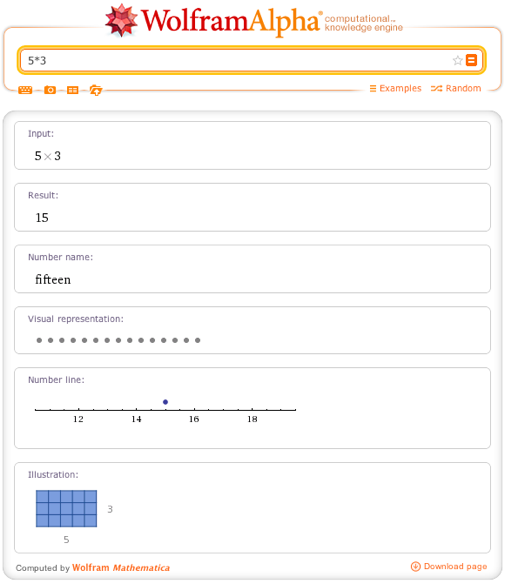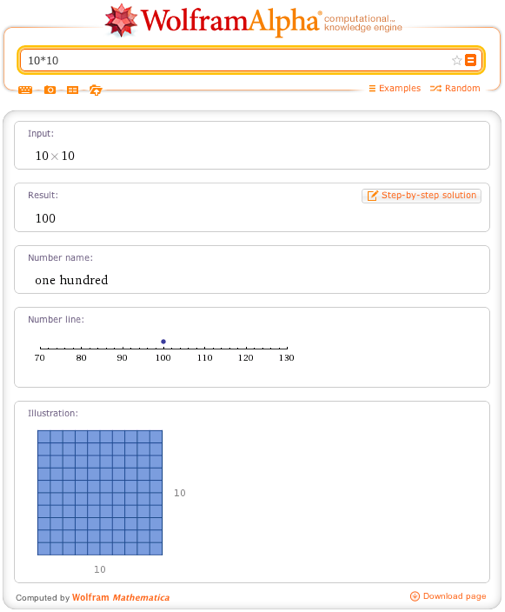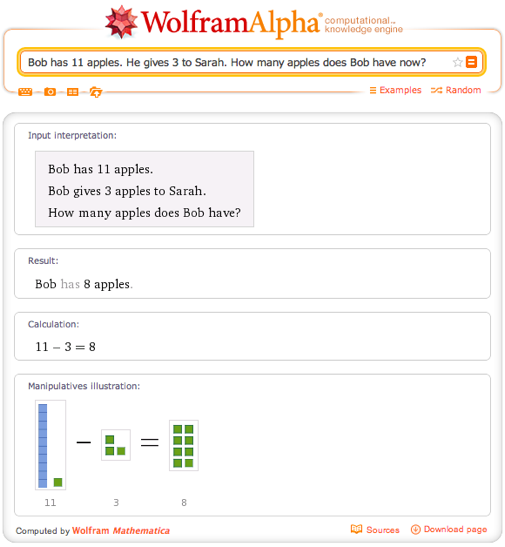# Math for All Ages—Online Manipulatives for Basic Arithmetic

January 15, 2013 —Comments Off

The Wolfram|Alpha math team adds new and exciting content to Wolfram|Alpha on a daily basis! In fact, over the past few months we’ve added a wide range of features and we will be introducing them in a blog series here. Lately, we’ve made an effort to make Wolfram|Alpha a powerful learning tool for those learning arithmetic! If you are either teaching or learning addition, multiplication, or basic math word problems, Wolfram|Alpha can help you.As you can see, users can follow the number line to see that 4 + 7 is 11, or they can count the Manipulatives in the pod below.

The number line is especially useful when you are adding negative numbers.You can even add (or subtract) three or more numbers. Let’s check it out:Of course, Wolfram|Alpha can also help with adding and subtracting fractions. How about 3/4 + 2/3?In this case, you can use the pie charts to help visualize what this problem really means. You can also use the Step-by-step button to see the steps to adding these fractions.

Don’t worry, if you are already learning multiplication, we’ve also added some visuals for you!In the Illustration pod, you can see that a 5 by 3 rectangle contains 15 boxes. Of course you can do this with bigger numbers as well.You might not want to sit there and count the boxes, but I promise, there are 100 little squares in a 10 by 10 square. Of course, in this example, you also have the ability to see the steps for multiplication of 10*10.

And if you have mastered simple arithmetic and are working toward word problems, we have support for simple word problems as well. Let’s check out the following example: Bob has 11 apples. He gives 3 to Sarah. How many apples does Bob have now?Once you have become a pro at arithmetic and word problems, don’t worry, Wolfram|Alpha’s math support goes FAR beyond this. Be sure to continue exploring Wolfram|Alpha for all of your math help!

where do the students manipulate? Or are the “Manipulatives” just pictures of objects?

Posted by Sue Jones January 15, 2013 at 6:53 pm

Thanks for the comment. What we show in our results are illustrations of manipulatives. Showing the results in this style help visual learners understand the concepts.

Posted by The Wolfram|Alpha Team January 30, 2013 at 9:12 am

I’m thrilled with your beginning efforts, but many student thinking problems must be addressed, which of course, is impossible to address automatically all problems in thinking.
But, If you permit why responses from students for confusing steps and confusing results, addressing only the why questions that come up, I believe you will come even closer to a perfect math tutor. e.g. your pie chart example of addition of fractions left the yellow shaded regions of the fractions unclear how they were combined to arrive at the final shaded region.

Posted by JOHN L HANK January 26, 2013 at 6:41 pm

I agree. Insert a 9/12 + 8/12 in between the problem and the answer.

Posted by John Plencner June 1, 2013 at 12:17 pm

I hated math growing up and found a love of it as I grew older. Give her puzzles and logic problems, but let her dictate what she wants. She will let you know what she likes. Just support her as best you can without pushing. The subject is way more interesting then what is taught.

Posted by Hanah October 7, 2014 at 3:58 am

This is very easy for children to understand, I sure wouldn’t of minded having such a helpful hand learning math of all subjects. Keeping it simple whilst demonstrating how the answer is reached is the best way possible.

Posted by domestic cleaning poole December 9, 2014 at 4:42 am